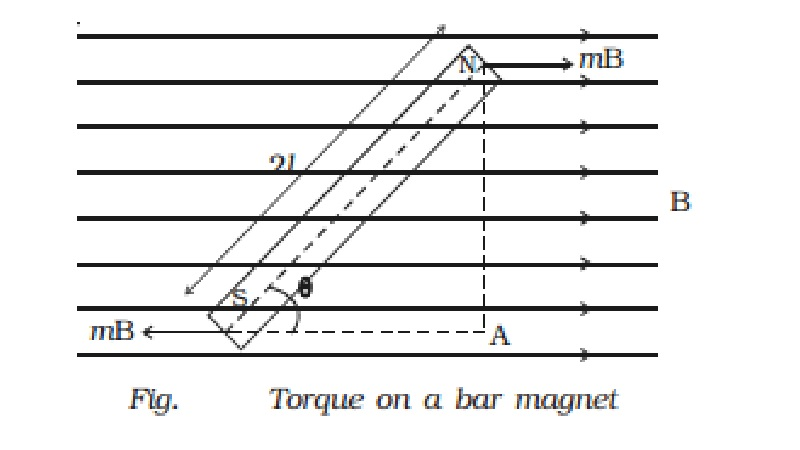Home | | Physics | | Physics | Torque on a bar magnet placed in a uniform magnetic field

# Torque on a bar magnet placed in a uniform magnetic fieldConsider a bar magnet NS of length 2l and pole strength m placed in a uniform magnetic field of induction B at an angle θ with the direction of the field (Fig.).

Torque on a bar magnet placed in a uniform magnetic field

Consider a bar magnet NS of length 2l and pole strength m placed in a uniform magnetic field of induction B at an angle θ with the direction of the field (Fig.).Due to the magnetic field B, a force mB acts on the north pole along the direction of the field and a force mB acts on the south pole along the direction opposite to the magnetic field.

These two forces are equal and opposite, hence constitute a couple.

The torque τ due to the couple is

τ = one of the forces ? perpendicular distance between them

τ = F ? NA

= mB ? NA ...(1)

= mB ? 2l sin θ

τ = MB sin θ ...(2)

Vectorially

Vec τ = Vec  M ? Vec  B

The direction of τ is perpendicular to the plane containing Vec M and  Vec B.

If B = 1 and θ = 90o

Then from equation (2),   τ  = M

Hence, moment of the magnet M is equal to the torque necessary to keep the magnet at right angles to a magnetic field of unit magnetic induction.

Study Material, Lecturing Notes, Assignment, Reference, Wiki description explanation, brief detail

Related Topics